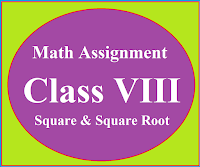## Posts

Showing posts from January, 2020

### Math Assignment Class VIII | Square & Square RootMath Assignment  Class VIII | Square & Square Root Download or Print free  assignment with answer key  for   Class  8 Squares and  Square Roots.   Important and extra questions that cover all topics of square and square root and is useful and helpful for the students. Math Assignment  Class VIII | Square & Square Root LEVEL -1

### Math Assignment Ch-12 Class X | Area Related to Plane FiguresMATHEMATICS ASSIGNMENT CLASS 12 Area related to Plane Figures Math Assignment Class X : Chapter 12 : Area Related to Plane Figures like triangle, quadrilaterals and circle etc. Area of minor sector, major sector, minor segment , major segment, semi-circle, quadrants etc. **************************************************************** This assignment is very useful for the students of class X who want to get full knowledge about the concept. It is necessary for the students, to study NCERT book first then examples of NCERT and then go through this assignment. This procedure provides you the best results in the examination. Question 1 Area of sector of circle of radius 36 cm is 54π cm 2  . Find the length of the corresponding arc of the sector. [Answer 3π]   Question 2 The wheel of the motorcycle is of radius 35 cm. How many revolutions per minute must the wheel make so as to keep a speed a speed of 66 km/h [Answer 500]   Question 3 A cow is tied with a rope of

### Theorem Class 10 Ratio of Area of two similar trianglesTheorem 6.6 class 10 mathematics, theorem based on the ratio of area of two similar triangles, theorem based on the relationship between ratio of areas and the corresponding sides. Statement :  The ratio of the areas of two similar triangles is equal to the square of the ratio of their corresponding sides. Given :-   $\Delta ABC\sim \Delta PQR$ To Prove :- $\frac{Ar(\Delta ABC)}{Ar(\Delta PQR)}=\left ( \frac{AB}{PQ} \right )^{2}=\left ( \frac{BC}{QR} \right )^{2}=\left ( \frac{AC}{PR} \right )^{2}$ Construction :-      $Draw\: \: AM\perp BC\: \: and\: PN\perp QR$ Proof :-      $\Delta ABC\sim \Delta PQR$ $\therefore \: \: \frac{AB}{PQ}=\frac{BC}{QR}=\frac{AC}{PR}......(1)$   $and\: \: \angle A=\angle P,\: \angle B=\angle Q\: ,\: \angle C=\angle R$ $Area\: of\: \Delta ABC=\frac{1}{2}\times BC\times AM$ $Area\: of\: \Delta PQR=\frac{1}{2}\times QR\times PN$ \[\frac{Ar(\Delta ABC)}{Ar(\Delta PQR)}=\frac{\frac{1}{2}\times BC\times AM}{\frac{1

### Techniques To Make E - Lesson PlanTechniques To Make E - Lesson Plan For Teachers Method to write effective e - lesson plan for teachers, Method of making lesson plan in mathematics Effective lesson plan step by step, method to make e lesson plan with hyperlinks. CBSE Format for Making E-Lesson Plan For the Teachers : In March, 2021, CBSE issued a " Technology Integration Lesson Plan Template_5Es" This Template  is very useful and helpful to the teachers in making E-Lesson Plan. Teachers can easily use this Template for making E-Lesson Plan. Click Here To download this Template in the word form. Teaching is the profession which teach all other professions. Students can follow the teaching only if it is interesting, systematic and effective. In order to achieve all these aspects of teaching a teacher should plan what he is going to deliver. Planning made by the teacher to deliver his lesson is called lesson plan. Lesson Plan Lesson plan is the planning made by the teacher to  make teaching learning pr